# linear algebra Determine whether the function is a linear transformation. T: R2 R3, T(x, y) =...

linear algebra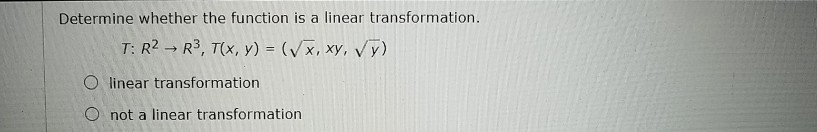Determine whether the function is a linear transformation. T: R2 R3, T(x, y) = (x,xy, vy) O linear transformation O not a linear transformation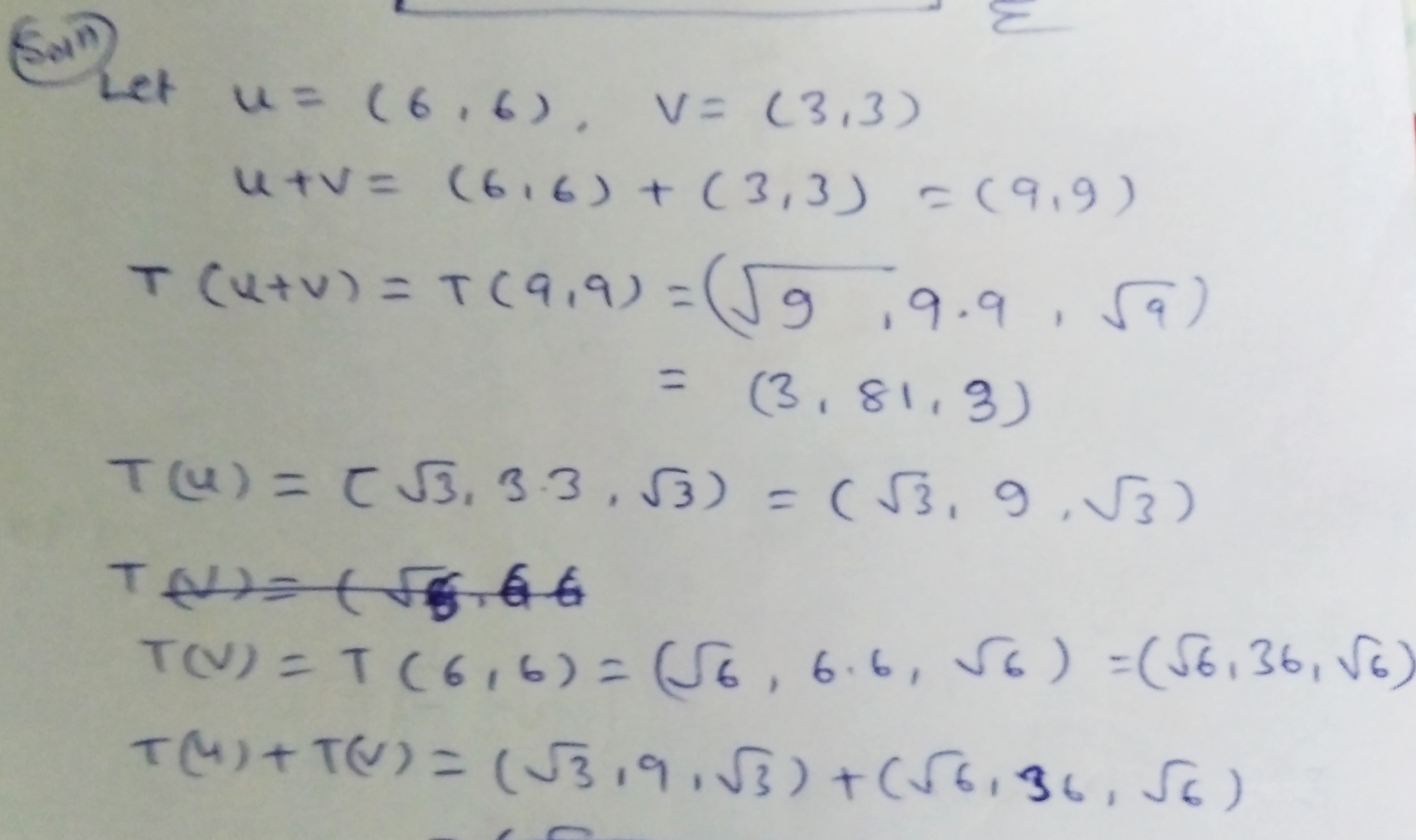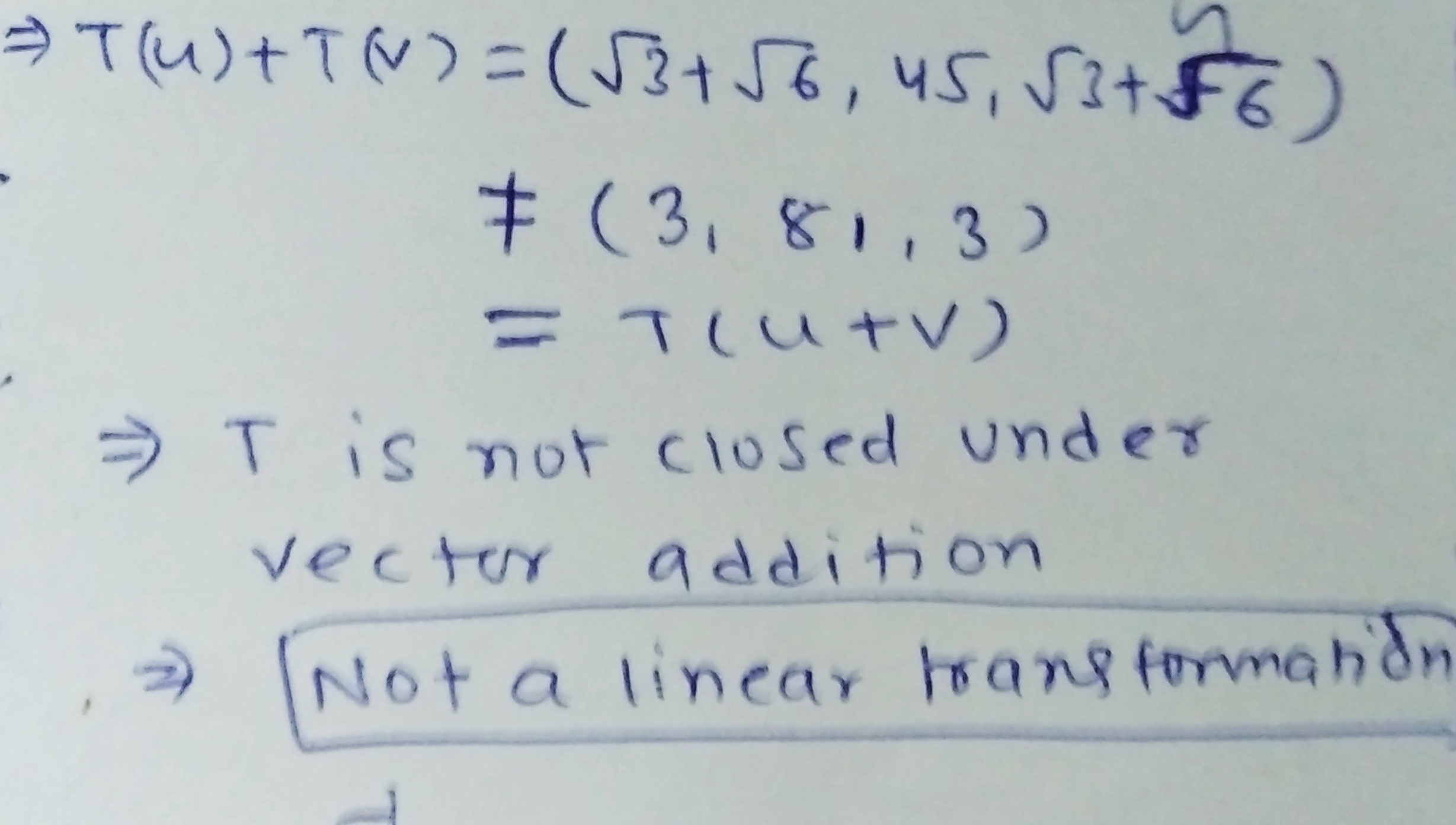##### Add Answer of: linear algebra Determine whether the function is a linear transformation. T: R2 R3, T(x, y) =...
Similar Homework Help Questions
• ### Determine whether the function is a linear transformation. T: R2 R3, T(x,y) = (Vx, 5xy, vy)...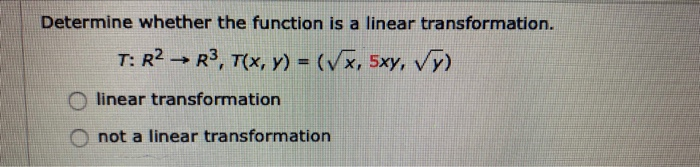Determine whether the function is a linear transformation. T: R2 R3, T(x,y) = (Vx, 5xy, vy) O linear transformation not a linear transformation

• ### DETAILS LARLINALG8 6.R.013. Determine whether the function is a linear transformation. T: R2 – R2, T(x,...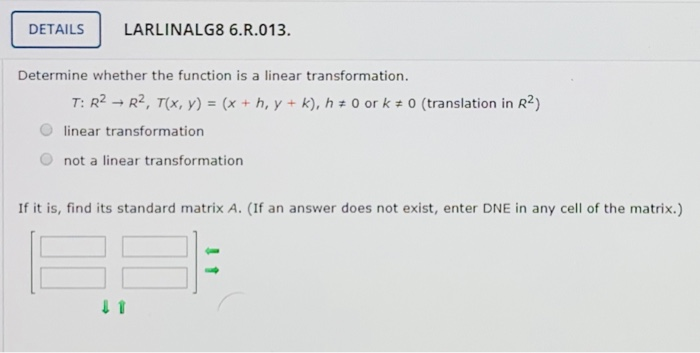DETAILS LARLINALG8 6.R.013. Determine whether the function is a linear transformation. T: R2 – R2, T(x, y) = (x + h. y + k), h + 0 or k + 0 (translation in R2) linear transformation not a linear transformation If it is, find its standard matrix A. (If an answer does not exist, enter DNE in any cell of the matrix.) 11

• ### By justifying your answer, determine whether the function T is a linear transformation. (a) T :...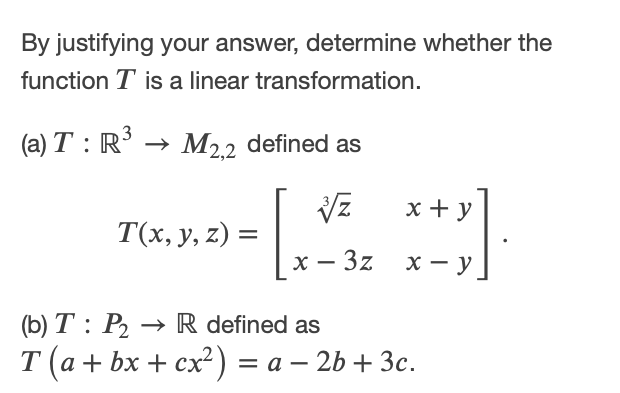By justifying your answer, determine whether the function T is a linear transformation. (a) T : R3 → M2,2 defined as x+y T(x, y, z) = x – 3z x - y (b) T : P2 → R defined as T (a + bx + cx?) = a – 2b + 3c. +

• ### Let T: R3 → R2 T(x, y, z) = (x + y,y+z) a. Is T a...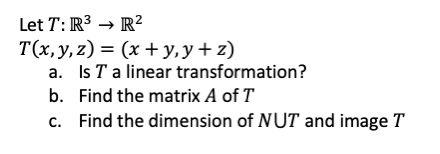Let T: R3 → R2 T(x, y, z) = (x + y,y+z) a. Is T a linear transformation? b. Find the matrix A of T C. Find the dimension of NUT and image T

• ### linear algebra Let T: R3 R3 be a linear transformation. Use the given information to find...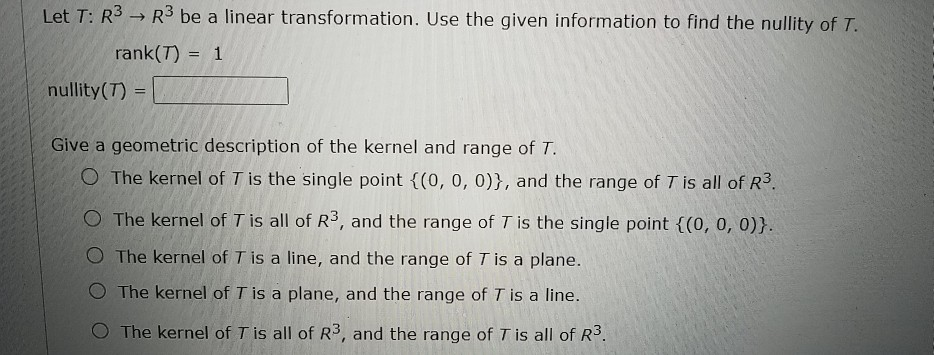linear algebra Let T: R3 R3 be a linear transformation. Use the given information to find the nullity of T. rank(T) = 1 nullity(T) = Give a geometric description of the kernel and range of T. The kernel of T is the single point {(0, 0, 0)}, and the range of T is all of R3. O The kernel of T is all of R3, and the range of T is the single point {(0, 0, 0)}. The kernel of...

• ### Let T: R2 + R2 be a linear transformation with PT(x) = 22 – 1. Determine/Compute...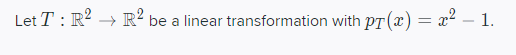Let T: R2 + R2 be a linear transformation with PT(x) = 22 – 1. Determine/Compute the linear transformation T2 : R2 + R2, vH T(T(v)). Show all your work for full credit.

• ### Is the transformation, T, given below a Linear Transformation where T: R2 -> R2 [:] -...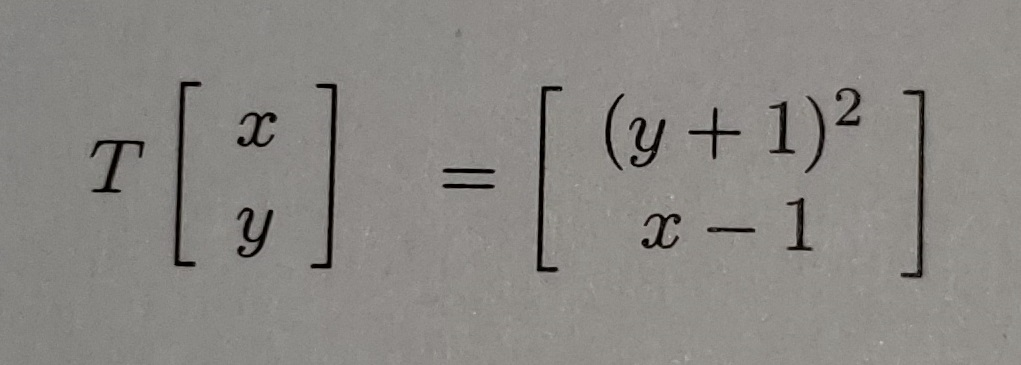Is the transformation, T, given below a Linear Transformation where T: R2 -> R2 [:] - [+*] (y + 1)2 1 x - 1 1

• ### linear algebra Let T: R2 R2 be a reflection in the line y = -x. Find...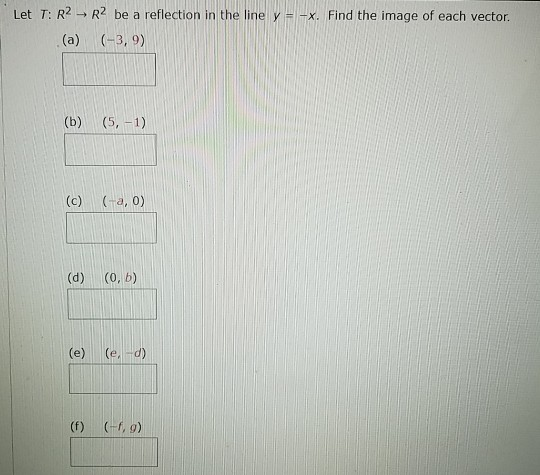linear algebra Let T: R2 R2 be a reflection in the line y = -x. Find the image of each vector. (a) (-3,9) (b) (5, -1) (c) (a,0) (d) (o, b) (e) ( ed) (f) (9)

• ### Q8 6 Points Let T : R2 + Rº be a linear transformation with PT(x) =...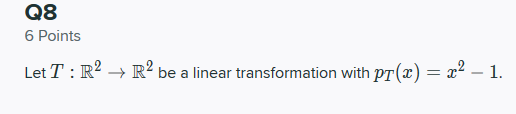Q8 6 Points Let T : R2 + Rº be a linear transformation with PT(x) = x2 – 1. Decide whether or not such a T is always diagonalizable. Justify your answer.. Q8.2 3 Points Determine/Compute the linear transformation T2 : R2 + R2, VH T(T(u)).

• ### Consider the linear transformation T: R3 + R2 defined as T(X1, X2, 23)=(-23, -3 &1 –...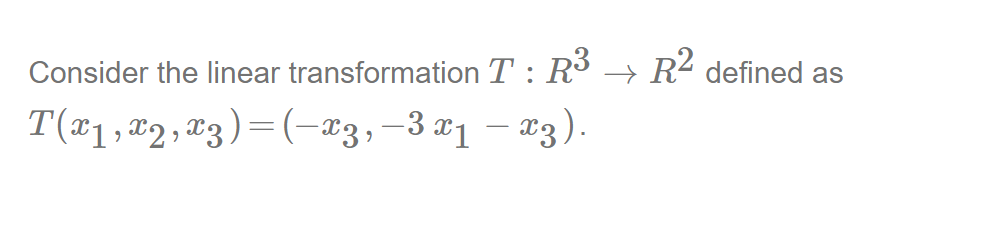Consider the linear transformation T: R3 + R2 defined as T(X1, X2, 23)=(-23, -3 &1 – 23). Write the standard matrix for HoT, where H is the reflection of R2 about the y-axis. ab sin (a) a дх f a 12 ?

Free Homework App